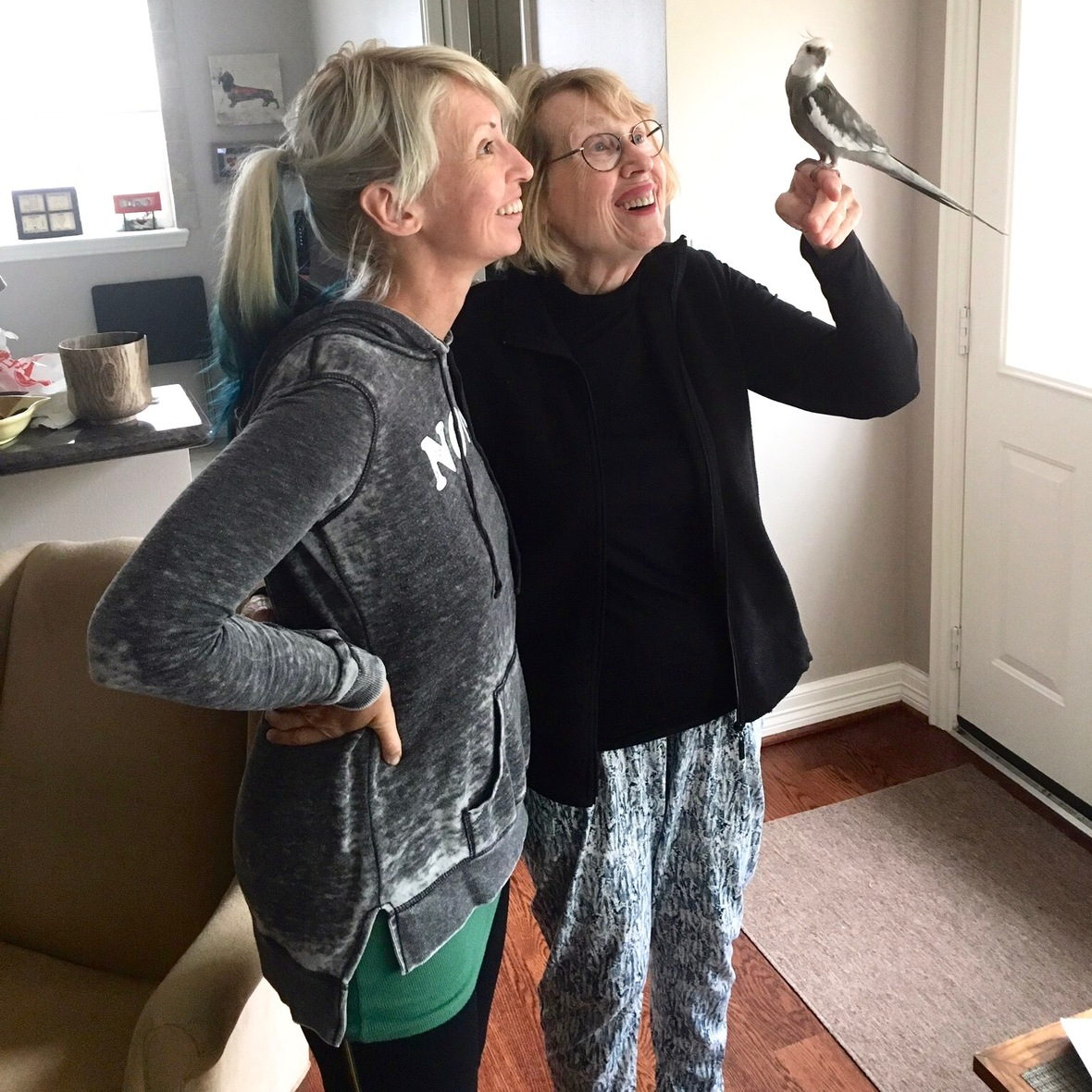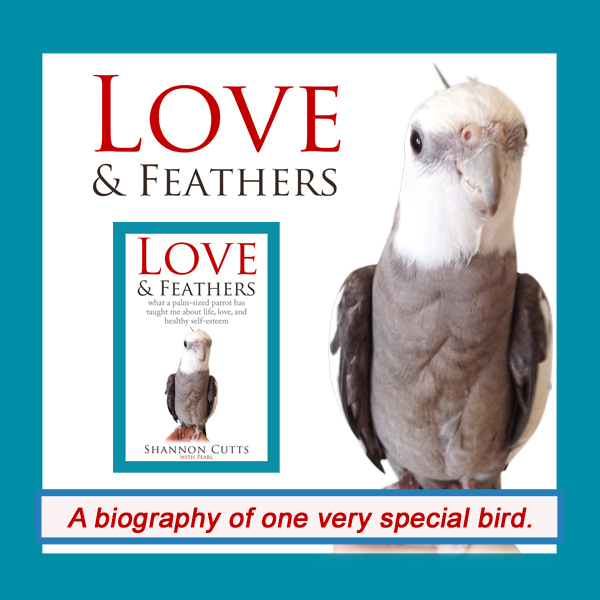# June 2019

 table div table+table+table div table{width:100%;padding:0}table div table+table+table div table img{width:96.23%;padding:0;float:none}table div table+table+table div table td{width:100%;padding:0 1.88% 18px}/* styles *//* styles */ Receiving this email as a forward? Sign up to keep following our little flock's adventures!
 table div table+table+table+table+table+table div table{width:100%;padding:0}table div table+table+table+table+table+table div table img{width:96.23%;padding:0;float:none}table div table+table+table+table+table+table div table td{width:100%;padding:0 1.88% 18px}/* styles */## Getting Comfortable in Your Own CompanyIf there is one flock member who definitely prefers to be surrounded by his peeps at all time, it is Pearl. Alone time? No thanks!

Over the last several months I have logged a significant amount of time alone with myself.

As a natural introvert, when I say I've spent a lot of time alone, I mean a LOT.

The other day, I was telling a dear friend how I hadn't left my casa for three straight days and nights - not even venturing as far as the end of the driveway (although Pearl and I had spent many happy hours basking in the sun on our little treehouse porch).

She expressed....shock. And that is when I felt it. SHAME.

Shame for spending three back-to-back days of time with only me.

I sincerely doubt my friend intended to shame me. No, this was an inside job. Her words touched some bruised hidden bit within me that reacted with an instant snap, snap, snap of its sharp, ashamed little teeth.

The day I shared this with my friend, I had at last ventured out to run errands, shop for groceries, do a bit of judicious thrifting, that sort of thing. Everywhere I went, everyone I saw seemed absorbed in....a certain small rectangular handheld object.

They were so intent! So focused! The day after that I ventured out again, this time to take a brisk walk at a favorite local park. What did I see? More of the same.

Did you know companies are now even making yoga pants with little phone-sized side-leg pockets? At the park I saw so many pairs of tiny earphones, delicate cables snaking down into those very side-leg pockets, as people walked, jogged, sprinted without ever once breaking their connection.

I realize I may be at risk here of reverse-shaming those people for using their phones, and I don't mean to - truly. It is a delicate balancing act and I struggle sometimes too. My point in sharing this is, to some degree, it feels like smart phones are no longer accessories but companions - to the point where leaving mine behind in my car while I ventured out to walk the three-mile tree-lined trail felt, well, kind of lame, actually.

Where a generation or two prior to now, people might have occupied themselves while waiting at the bar or at a traffic light by lighting a cigarette, today we automatically (me included) grab that spare moment to check in with the small rectangular sidekick nestled in our bag or back pocket.

Sure, the whole three days I spent inside my house I was working at my regular job, writing freelance articles for various clients, and of course checking in with my phone at regular intervals. Honestly, I might as well have told my friend I just stayed overnight for three nights and slept at the office, especially since in a weird sort of way it is actually true.

But the main reason I didn't venture out of my casa wasn't because I didn't want to or didn't need to. It was because I chose to stay in.

I did let some errands pile up in the interests of keeping myself close to home. I spent those three days deliberately paying attention to some of the ways I let my restless mind and aching heart talk me into scattering my focus and energy here and there, on this and that, so I won't have to feel that awful, uncomfortable inner squirm that arises when I turn my attention back towards my aching, healing self.

I can't remember which of my many beloved mentors and teachers (Pema Chodron, perhaps?) talked about how we come into this world alone and we die alone - those are two of the most significant experiences any being ever has in the course of life.

This - along with a still-fresh parting from my longtime love a handful of months ago - woke me up to the state of my connection with me. I realized, if I am going to be the one who sits by my own bedside, who holds my own hand, who listens to unspoken yet heartfelt confidences as I take my final breaths, well, I want to be my best friend.

I want to be so comfortable with me, so familiar with me, so accepting of me, so kindly disposed towards me, so loving towards me, so at home in my own presence, that I don't spend those last weeks or days flailing about for "someone else" to accompany me. And I don't want to feel ashamed if I don't have anyone else other than me who wants the job.

Truly, I want to accompany myself. And I want to do so with gratitude and grace, honor and humility, joy and love.

To do this, I must spend time with me. I must befriend me. More than that, I really WANT to.

with great love and respect,

Shannon

 table div table+table+table+table+table+table+table+table+table div table{width:100%;padding:0}table div table+table+table+table+table+table+table+table+table div table img{width:96.23%;padding:0;float:none}table div table+table+table+table+table+table+table+table+table div table td{width:100%;padding:0 1.88% 18px}/* styles *//* styles */ Do you feel mostly comfortable in your own presence? Does your own solitary company fill you with ease? Or do you feel uncertainty, discomfort, anxiety or perhaps depression at the thought of an un-filled calendar and unscheduled alone-time? There is no doubt we are all wired different - I, for example, am a stark raving introvert, while my beloved mom is just the opposite. But these temperament contrasts aside, do you feel you develop more comfort, more "at-homeness" with your own company as the years pass? I'd love to hear your stories!
 table div table+table+table+table+table+table+table+table+table+table+table div table{width:100%;padding:0}table div table+table+table+table+table+table+table+table+table+table+table div table img{width:96.23%;padding:0;float:none}table div table+table+table+table+table+table+table+table+table+table+table div table td{width:100%;padding:0 1.88% 18px}/* styles */# "Waffles & More" - Order Your Tasty Copy Today!

 table.module-12{width:75.47%;padding:0}table div table+table+table+table+table+table+table+table+table+table+table+table+table div table{width:75.47%;float:none;margin-left:auto;margin-right:auto;padding:0}table div table+table+table+table+table+table+table+table+table+table+table+table+table div table a{border:0 none;text-decoration:none}table div table+table+table+table+table+table+table+table+table+table+table+table+table div table img{width:100%!important;border:0 none;text-decoration:none}table div table+table+table+table+table+table+table+table+table+table+table+table+table div table td{width:100%;padding:0}/* styles */
 table div table+table+table+table+table+table+table+table+table+table+table+table+table+table+table div table{width:100%;padding:0}table div table+table+table+table+table+table+table+table+table+table+table+table+table+table+table div table img{width:96.23%;padding:0;float:none}table div table+table+table+table+table+table+table+table+table+table+table+table+table+table+table div table td{width:100%;padding:0 1.88% 18px}/* styles */# Get your copy of Love & Feathers the book!Click on the image of Pearl to order your signed copy!Pearl will even beak-o-graph it for you!
 table div table+table+table+table+table+table+table+table+table+table+table+table+table+table+table+table+table+table+table+table div table{width:100%;padding:0}table div table+table+table+table+table+table+table+table+table+table+table+table+table+table+table+table+table+table+table+table div table img{width:96.23%;padding:0;float:none}table div table+table+table+table+table+table+table+table+table+table+table+table+table+table+table+table+table+table+table+table div table td{width:100%;padding:0 1.88% 18px}/* styles */# Guess who got stuck in the....turtle slipper?

 table.module-21{width:75.47%;padding:0}table div table+table+table+table+table+table+table+table+table+table+table+table+table+table+table+table+table+table+table+table+table+table div table{width:75.47%;float:none;margin-left:auto;margin-right:auto;padding:0}table div table+table+table+table+table+table+table+table+table+table+table+table+table+table+table+table+table+table+table+table+table+table div table a{border:0 none;text-decoration:none}table div table+table+table+table+table+table+table+table+table+table+table+table+table+table+table+table+table+table+table+table+table+table div table img{width:100%!important;border:0 none;text-decoration:none}table div table+table+table+table+table+table+table+table+table+table+table+table+table+table+table+table+table+table+table+table+table+table div table td{width:100%;padding:0}/* styles */
 table div table+table+table+table+table+table+table+table+table+table+table+table+table+table+table+table+table+table+table+table+table+table+table+table div table{width:100%;padding:0}table div table+table+table+table+table+table+table+table+table+table+table+table+table+table+table+table+table+table+table+table+table+table+table+table div table img{width:96.23%;padding:0;float:none}table div table+table+table+table+table+table+table+table+table+table+table+table+table+table+table+table+table+table+table+table+table+table+table+table div table td{width:100%;padding:0 1.88% 18px}/* styles */# Our dashing and debonair Bruce!

 table.module-25{width:75.47%;padding:0}table div table+table+table+table+table+table+table+table+table+table+table+table+table+table+table+table+table+table+table+table+table+table+table+table+table+table div table{width:75.47%;float:none;margin-left:auto;margin-right:auto;padding:0}table div table+table+table+table+table+table+table+table+table+table+table+table+table+table+table+table+table+table+table+table+table+table+table+table+table+table div table a{border:0 none;text-decoration:none}table div table+table+table+table+table+table+table+table+table+table+table+table+table+table+table+table+table+table+table+table+table+table+table+table+table+table div table img{width:100%!important;border:0 none;text-decoration:none}table div table+table+table+table+table+table+table+table+table+table+table+table+table+table+table+table+table+table+table+table+table+table+table+table+table+table div table td{width:100%;padding:0}/* styles */
 table div table+table+table+table+table+table+table+table+table+table+table+table+table+table+table+table+table+table+table+table+table+table+table+table+table+table+table+table div table{width:100%;padding:0}table div table+table+table+table+table+table+table+table+table+table+table+table+table+table+table+table+table+table+table+table+table+table+table+table+table+table+table+table div table img{width:96.23%;padding:0;float:none}table div table+table+table+table+table+table+table+table+table+table+table+table+table+table+table+table+table+table+table+table+table+table+table+table+table+table+table+table div table td{width:100%;padding:0 1.88% 18px}/* styles */## Meet Pearl, Malti, Bruce & Me

 table div table+table+table+table+table+table+table+table+table+table+table+table+table+table+table+table+table+table+table+table+table+table+table+table+table+table+table+table+table+table div table,table.module-29{width:34.34%;float:left;padding:0}table div table+table+table+table+table+table+table+table+table+table+table+table+table+table+table+table+table+table+table+table+table+table+table+table+table+table+table+table+table+table div table a{border:0 none;text-decoration:none}table div table+table+table+table+table+table+table+table+table+table+table+table+table+table+table+table+table+table+table+table+table+table+table+table+table+table+table+table+table+table div table img{width:100%!important;border:0 none;text-decoration:none}table div table+table+table+table+table+table+table+table+table+table+table+table+table+table+table+table+table+table+table+table+table+table+table+table+table+table+table+table+table+table div table td{width:100%;padding:0 20px 20px 0}/* styles */ Hi! It is so good to meet you here each month! I'm so excited about our newest book, "Waffles & More: A Love & Feathers Recipe Book," which features so many of the recipes our flock (and especially the flock member with the feathers) particularly enjoys! And of course there is the book that started it all - "Love & Feathers: what a palm-sized parrot has taught me about life, love, and healthy self-esteem." This fun and feathery book focuses on the power of animal mentors in our lives and features my life with my precious parrot, Pearl. Malti is my darling young red-foot tortoise. She has such a healthy appetite for food, snoozing and life! Bruce, a very brave rescued Texas 3-toed box turtle, joined our flock a few years ago in a space clearly reserved just for him! My first book, "Beating Ana: how to outsmart your eating disorder and take your life back," is a practical hands-on workbook to help overcome eating disorders as well as the story of my personal recovery journey. MentorCONNECT, the first global eating disorders nonprofit mentoring community, emerged from the experience of writing "Beating Ana." While we closed in April 2017, the website continues to provide information about mentoring-based recovery support resources. ==> To connect with me: www.shannoncutts.com ==> To connect with Pearl, Malti & Bruce: www.loveandfeathersandshells.com
 table div table+table+table+table+table+table+table+table+table+table+table+table+table+table+table+table+table+table+table+table+table+table+table+table+table+table+table+table+table+table+table div table{width:100%;padding:0}table div table+table+table+table+table+table+table+table+table+table+table+table+table+table+table+table+table+table+table+table+table+table+table+table+table+table+table+table+table+table+table div table img{width:96.23%;padding:0;float:none}table div table+table+table+table+table+table+table+table+table+table+table+table+table+table+table+table+table+table+table+table+table+table+table+table+table+table+table+table+table+table+table div table td{width:100%;padding:0 1.88% 18px}/* styles */table div table+table+table+table+table+table+table+table+table+table+table+table+table+table+table+table+table+table+table+table+table+table+table+table+table+table+table+table+table+table+table+table div table td,table.module-31{width:100%;padding:0}table div table+table+table+table+table+table+table+table+table+table+table+table+table+table+table+table+table+table+table+table+table+table+table+table+table+table+table+table+table+table+table+table div table{width:100%;float:none;margin-left:auto;margin-right:auto;padding:0}table div table+table+table+table+table+table+table+table+table+table+table+table+table+table+table+table+table+table+table+table+table+table+table+table+table+table+table+table+table+table+table+table div table a{border:0 none;text-decoration:none}table div table+table+table+table+table+table+table+table+table+table+table+table+table+table+table+table+table+table+table+table+table+table+table+table+table+table+table+table+table+table+table+table div table img{width:100%!important;border:0 none;text-decoration:none}/* styles */
 table.module-32{width:74.15%;padding:0}table div table+table+table+table+table+table+table+table+table+table+table+table+table+table+table+table+table+table+table+table+table+table+table+table+table+table+table+table+table+table+table+table+table div table{width:74.15%;float:none;margin-left:auto;margin-right:auto;padding:0}table div table+table+table+table+table+table+table+table+table+table+table+table+table+table+table+table+table+table+table+table+table+table+table+table+table+table+table+table+table+table+table+table+table div table a{border:0 none;text-decoration:none}table div table+table+table+table+table+table+table+table+table+table+table+table+table+table+table+table+table+table+table+table+table+table+table+table+table+table+table+table+table+table+table+table+table div table img{width:100%!important;border:0 none;text-decoration:none}table div table+table+table+table+table+table+table+table+table+table+table+table+table+table+table+table+table+table+table+table+table+table+table+table+table+table+table+table+table+table+table+table+table div table td{width:100%;padding:0}/* styles */
 table div table+table+table+table+table+table+table+table+table+table+table+table+table+table+table+table+table+table+table+table+table+table+table+table+table+table+table+table+table+table+table+table+table+table div table{width:100%;padding:0}table div table+table+table+table+table+table+table+table+table+table+table+table+table+table+table+table+table+table+table+table+table+table+table+table+table+table+table+table+table+table+table+table+table+table div table img{width:96.23%;padding:0;float:none}table div table+table+table+table+table+table+table+table+table+table+table+table+table+table+table+table+table+table+table+table+table+table+table+table+table+table+table+table+table+table+table+table+table+table div table td{width:100%;padding:0 1.88% 18px}/* styles */## Reprints & Reposts

REPRINTS: Would you like to use any portion of this material in your newsletter, blog or website? You may do so as long as you also include this copyright information: